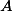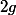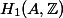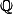# Martin Orr's Blog

## Period relations on abelian varieties

Posted by Martin Orr on Monday, 26 October 2015 at 11:00

The Legendre period relation is a classical equation relating the periods and quasi-periods of an elliptic curve, as defined last time. I will discuss this relation, and then more generally discuss how the existence of polarisations implies relations between the periods of higher-dimensional abelian varieties.

These examples motivate the introduction of the geometric motivic Galois group, which gives an upper bound for the transcendence degree of periods of an abelian variety (or indeed any algebraic variety). This upper bound is conjectured to be equal to the actual transcendence degree. I had intended to discuss the geometric motivic Galois group in this post too, but I decided that it was getting to long so I will postpone that to another time.

## Periods of abelian varieties

Posted by Martin Orr on Tuesday, 06 October 2015 at 16:10

There are a couple of different matrices associated with an abelian variety which are referred to as its period matrix. These matrices relate different choices of bases for the tangent space or `` of the abelian variety. In this post I will discuss the different definitions of period matrices and how they relate to each other.

A complex abelian variety `` can be realised as the quotient of the-dimensional complex vector space `` by the rank-lattice ``. The period matrix expresses a basis of this lattice in terms of a basis for the tangent space. We can also get a period matrix which is twice as large by using de Rham cohomology `` instead of the tangent space ``.

The period matrix can be defined for any complex abelian variety, but it contains additional information if the abelian variety is defined over a number field, as we can then choose our basis for `` to also be defined over. The period matrix then gives us a set of complex numbers relating a basis of a-vector space to a basis of a-vector space, and the transcendence properties of these numbers are interesting and I will discuss them in a later post.